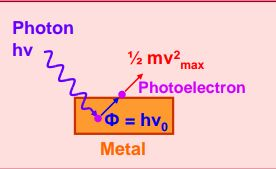#### When photons of wavelength λ1 are incident on an isolated sphere, the corresponding stopping potential is found to be V.  When photons of wavelength  λ2 are used, the corresponding stopping potential was thrice that of the above value. If light of wavelength  λ3 is used then find the stopping potential for this case : Option 1)Option 2)Option 3)Option 4)As we learnt in

Conservation of energy -- whereinApplying Einstein photo electric equation.............(1).................(2)

Put wave of eV from equation (1) to equation (2)If wavelengthis used equation becomeCorrect option is 3.

Option 1)This is incorrect option.

Option 2)This is incorrect option.

Option 3)This is the correct option.

Option 4)This is incorrect option.

#### perimeter